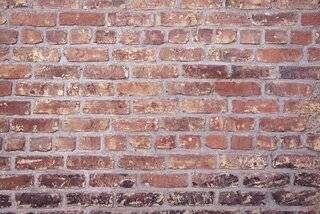# Brickwork rate analysis|3 materialsBrickwork rate analysis can be calculated by estimating materials cost ,labour cost,water charges and by adding contractor profits.

## Brickwork materials

Brickwork involves the following materials and labor cost.

1. Cement
2. Sand
3. Bricks

Let us consider length of brick wall as 10m and height as 3m and thickness of wall as 230mm.

Considering bricks of size 230mm x 115mm x 75mm (9” x 4.5” x 3”) were utilized.

### Estimating materials quantity

Volume of brick wall=10m x 3m x .23m=6.9 m3

Volume of 1 brick with out mortar=0.23m x0.115m x 0.075m=0.00198 m3

Volume of 1 brick with mortar=(0.23+0.01)m x (0.115+0.01) x (0.075+0.01) =0.24×0.125×0.085=0.00255m3, Considering 10mm thick mortar.

So total number of bricks required for 6.9 m3 volume brick wall=6.9/0.00255=2706 No’s.

Volume of 2706 No’s bricks with out mortar =2706 x0.00198=5.35 m3

Note: Volume of brick wall =Dry volume of bricks + volume of wet mortar

Volume of wet mortar = Volume of brick wall -Dry volume of bricks=6.9 m3-5.35m3=1.55m3

Note: Volume of dry mortar=1.33 x Volume of wet mortar

Volume of dry mortar=1.33 x 1.55 m3=2.06 m3

#### Quantity and Cost of Cement

So Volume of dry mortar for 6.9 m3 brick wall =2.06 m3

Considering cement ,sand ratio in brick wall is 1:4,Density of cement =1440 kg/m3

Quantity of Cement =2.06 x 1×1440/(1+4) =593.28 kgs =11.86 bags =12 bags

Considering cost of 1 cement bag= Rs 350.

So cost of 12 bags =350 x 12 =Rs 4200

#### Quantity and cost of Sand

Quantity of sand =2.06 x 4×1920/(1+4)=3165 kgs, Considering density of sand =1920kg/m3

Considering cost of sand is Rs 1600/MT

Cost of sand =3.165 MT x 1600 =Rs 5064

#### Cost of bricks

So 6.9m3 brickwall,2706 Nos red bricks are required.

Considering rate of 1 brick is Rs 7 ,So cost of 2706 bricks =2706 x 7 =Rs 18942

So Total cost of brick work =Cost of Cement + Cost of Sand + Cost of Bricks

= Rs 4200 + Rs 5064 + 18942

=Rs 28206

Labour Cost

For 1m3 1 mason and 1 helper is required

Assuming Cost of 1 mason is Rs 800 and cost of 1 helper is Rs 500

So for 6.9 m3 of brick work, nearly 7 masons and 7 helpers are required.

So cost of Labour =7×800 + 7×500=Rs 9100

So total cost of brickwork including labour =28206 +9100 =Rs 37306

### Cost of 1 cum brickwork

So cost of 6.9 m3 brick work is Rs 37306

Cost of 1m3 brick work =37306/6.9= Rs 5407

Add 10% contractor profit to total cost = Rs 5407x 0.10=Rs 540.7

So Total cost of 1m3 brickwork =Rs 5407 +Rs 541 =Rs 5948

Read More – 48 quick steps for house construction#### About Ashwini Kumar

Ashwini Kumar is a graduate in civil engineering from NIT Warangal. He has 8+ years of experience in the construction field. He is the author, founder of the Easy Home Builds blog

View all posts by Ashwini Kumar →